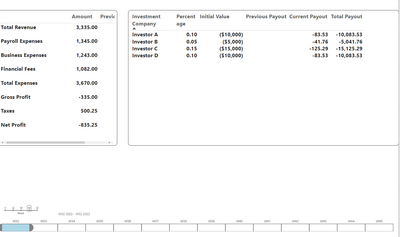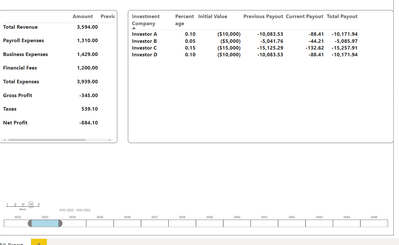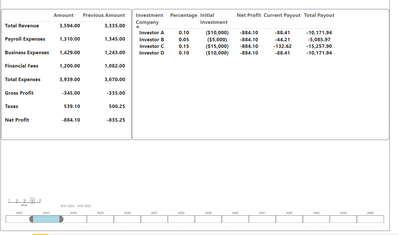cancel
Showing results for
Did you mean:Impactful Individual

## Accumulate calculated value over time

hellopowerbi community,
i have a dashboard i'm trying to build which includes  p/L report and next to it some investors payout.
i was able to build the P/L report with their value and previous period value.

now i want to build an accumulated table of payouts over time, i will share pic of how i want it to be but i played with the calculations just for the sake of showing you my desired results.

you can see first the inital week i want it like this:then if i select the next week i want it to look like this:you can find my dashboard here in the pbix file
https://1drv.ms/u/s!Ag9tIyk2ofNRjkNFD9Do7eDErauI?e=j6xXZ2

1 ACCEPTED SOLUTIONImpactful Individual

i finally found a solution for me and i will guide you through the steps :

first i created a  net profit measure

``````Net Profit =
VAR Totalrevenue = Calculate(SUM(Revenue[Amount]))
VAR Payroll = CALCULATE(SUM(Expenses[Amount]),FILTER(Expenses,Expenses[Category] = "Payroll Expenses"))
VAR Financial = CALCULATE(SUM(Expenses[Amount]),FILTER(Expenses,Expenses[Category] = "Financial Fees"))
Var Totalexpense = Calculate(SUM(Expenses[Amount]))
Var Grossprofit = (Totalrevenue - Totalexpense)
VAR Taxes = (Totalrevenue * 0.15)
VAR Netprofit = Grossprofit - Taxes
Return
Netprofit``````

later i created a "before" measure that calculates all net profit from my inital date to selected date in the following measure :

``````before = VAR seldate = MAX(DateTable[Date])
RETURN
CALCULATE([Net Profit],DATESBETWEEN(DateTable[Date],"8/1/2022",seldate))``````

Then i made my current payout which is

``````Current Payout =
[Net Profit] * MAX(Investors[Percentage])``````

and finally i have the total payout which is :

``Total Payout = ([before] * MAX(Investors[Percentage])) + MAX(Investors[Initial Value])``

here are some photos of my results :
for week 1 :for week 2:all is left is for me to create a previous payout measure which i already know how to solve half of it i just need a similar calcualtion to the "before" measure but he seldate is 1 week before can anyone help?

4 REPLIES 4Impactful Individual

i finally found a solution for me and i will guide you through the steps :

first i created a  net profit measure

``````Net Profit =
VAR Totalrevenue = Calculate(SUM(Revenue[Amount]))
VAR Payroll = CALCULATE(SUM(Expenses[Amount]),FILTER(Expenses,Expenses[Category] = "Payroll Expenses"))
VAR Financial = CALCULATE(SUM(Expenses[Amount]),FILTER(Expenses,Expenses[Category] = "Financial Fees"))
Var Totalexpense = Calculate(SUM(Expenses[Amount]))
Var Grossprofit = (Totalrevenue - Totalexpense)
VAR Taxes = (Totalrevenue * 0.15)
VAR Netprofit = Grossprofit - Taxes
Return
Netprofit``````

later i created a "before" measure that calculates all net profit from my inital date to selected date in the following measure :

``````before = VAR seldate = MAX(DateTable[Date])
RETURN
CALCULATE([Net Profit],DATESBETWEEN(DateTable[Date],"8/1/2022",seldate))``````

Then i made my current payout which is

``````Current Payout =
[Net Profit] * MAX(Investors[Percentage])``````

and finally i have the total payout which is :

``Total Payout = ([before] * MAX(Investors[Percentage])) + MAX(Investors[Initial Value])``

here are some photos of my results :
for week 1 :for week 2:all is left is for me to create a previous payout measure which i already know how to solve half of it i just need a similar calcualtion to the "before" measure but he seldate is 1 week before can anyone help?Super UserImpactful IndividualSuper User

your "Previous Payout" measure looks like it could use some cleanup.  Since you are already connecting to the dates table you could write it like this

VAR _max =
MIN ( 'DateTable'[Date] )
VAR _min =
2* _max -MAX ( 'DateTable'[Date] )

and then use that to project that filter onto the Dates table.

VAR Totalrevenue =
CALCULATE (
SUM ( Revenue[Amount] ),
FILTER ( ALL (DateTable[Date]), DateTable[Date]>=_min && DateTable[Date]<=_max)
)

Here is the full measure.

``````Previous Payout =
VAR _max =
MIN ( 'DateTable'[Date] )
VAR _min =
2* _max -MAX ( 'DateTable'[Date] )
VAR Totalrevenue =
CALCULATE (
SUM ( Revenue[Amount] ),
FILTER ( ALL (DateTable[Date]), DateTable[Date]>=_min && DateTable[Date]<=_max)
)
VAR Totalexpense =
CALCULATE (
SUM ( Expenses[Amount] ),
FILTER ( ALL (DateTable[Date]), DateTable[Date]>=_min && DateTable[Date]<=_max)
)
VAR Grossprofit = ( Totalrevenue - Totalexpense )
VAR Taxes = ( Totalrevenue * 0.15 )
VAR Netprofit = Grossprofit - Taxes
RETURN Netprofit``````

Hope this gets you closer to what you need.

Note that your Investors table is not connected to the data model.